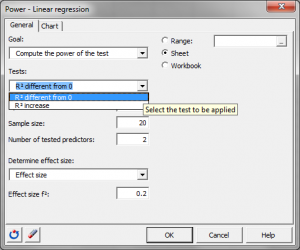# Statistical Power for linear regression

Ensure optimal power or sample size using power analysis. Power for linear regression is available in Excel using the XLSTAT statistical software.## Statistical Power for linear regression

XLSTAT-Pro offers a tool to apply a linear regression model. XLSTAT-Power estimates the power or calculates the necessary number of observations associated with variations of R ² in the framework of a linear regression.

When testing a hypothesis using a statistical test, there are several decisions to take:

• The null hypothesis H0 and the alternative hypothesis Ha.
• The statistical test to use.
• The type I error also known as alpha. It occurs when one rejects the null hypothesis when it is true. It is set a priori for each test and is 5%.

The type II error or beta is less studied but is of great importance. In fact, it represents the probability that one does not reject the null hypothesis when it is false. We cannot fix it up front, but based on other parameters of the model we can try to minimize it. The power of a test is calculated as 1-beta and represents the probability that we reject the null hypothesis when it is false.

We therefore wish to maximize the power of the test. The XLSTAT-Power module calculates the power (and beta) when other parameters are known. For a given power, it also allows to calculate the sample size that is necessary to reach that power.

The statistical power calculations are usually done before the experiment is conducted. The main application of power calculations is to estimate the number of observations necessary to properly conduct an experiment. XLSTAT allows you to compare:

• R² value to 0.
• Increase in R² value when new predictors are added to the model to 0.

It means testing the following hypothesis:

• H0: R² is equal to 0 / Ha: R² is different from 0
• H0: Increase in R² is equal to 0 / Ha: Increase in R² is different from 0.

## Effect size for the variation of R² in linear regression

This concept is very important in power calculations. Indeed, Cohen (1988) developed this concept. The effect size is a quantity that will allow you to calculate the power of a test without entering any parameters but will tell if the effect to be tested is weak or strong. In the context of a linear regression, conventions of magnitude of the effect size are:

• f²=0.02, the effect is small.
• f²=0.15, the effect is moderate.
• f²=0.35, the effect is strong.

XLSTAT-Power allows you to enter directly the effect size but also allows entering parameters of the model that will help calculating the effect size. We detail the calculations below:

• Using variances: We can use the variances of the model to define the size of the effect. With varExplained being the variance explained by the explanatory variables that we wish to test and varError being the variance of the error or residual variance, we have: f² = varExplained/ varError.
• Using the R² (in the case H0: R²=0): We enter the estimated square multiple correlation value (called rho²) to define the size of the effect. We have: f² = ρ² / (1 - ρ)
• Using the partial R² (in the case H0: Increase in R²=0): We enter the partial R² that is the expected difference in R² when adding predictors to the model to define the size of the effect. We have: f² = Rpart² / (1 - Rpart²)
• Using the correlations between predictors (in the case 0: R²=0): One must then select a vector containing the correlations between the explanatory variables and the dependent variable CorrY, and a square matrix containing the correlations between the explanatory variables CorrX. We have: f² = CorrYT * CorrX-1 * CorrY / (1 - = CorrYT * CorrX-1 * CorrY)

Once the effect size is defined, power and necessary sample size can be computed.

## Calculations of the Statistical Power for changes in R² in linear regression

The power of a test is usually obtained by using the associated non-central distribution. For this specific case we will use the Fisher non-central distribution to compute the power. The power of this test is obtained using the non-central Fisher distribution with degrees of freedom equal to: DF1 is the number of tested variables; DF2 is the sample size from which the total number of explanatory variables included in model plus one is subtracted and the non-centrality parameter is: NCP = f²N.

## Calculating sample size for changes in R² in linear regression

To calculate the number of observations required, XLSTAT uses an algorithm that searches for the root of a function. It is called the Van Wijngaarden-Dekker-Brent algorithm (Brent, 1973). This algorithm is adapted to the case where the derivatives of the function are not known. It tries to find the root of:

power (N) - expected_power

We then obtain the size N such that the test has a power as close as possible to the desired power.### analyze your data with xlstat

14-day free trial

Included in

Related features